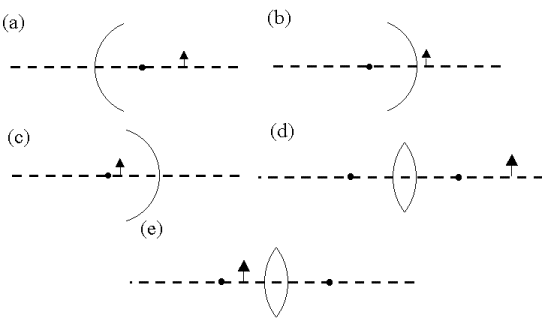Teaching Physics with the Physics Suite Edward F. Redish Problems Sorted by Type | Problems Sorted by Subject | Problems Sorted by Chapter in UP

Mirrors and lenses

Each of the problems in this part has a description of an object and an optical device (lens or mirror). A sketch is shown below. For each case, specify whether

• the image is real (R), virtual (V), or no image is formed (N) ;
• the image is on the same side of the device as the object (S) or the opposite side (O). If there is no image put a null mark ( f )
• if an image is formed, on which side of the system must the observer be in order to see it, left (-) or right (+)?
For each problem you should therefore give three answers (for example : V O +). For the mirrors the center is shown. For the lenses, the focal points are shown. The radius of curvature of the mirrors is R, the focal length of the lenses is f.

1. An object on the right side of a spherical mirror, a distance s > R from the mirror. The mirror is concave towards the object.
2. An object on the right side of a spherical mirror, a distance s < R/2 from the mirror. The mirror is convex towards the object.
3. An object on the left side of a spherical mirror, a distance R> s > R/2 from the mirror. The mirror is concave towards the object.
4. An object on the right side of a convex lens, a distance s > f from the lens.
5. An object on the left side of a convex lens, a distance s < f from the lensNot finding what you wanted? Check the Site Map for more information.